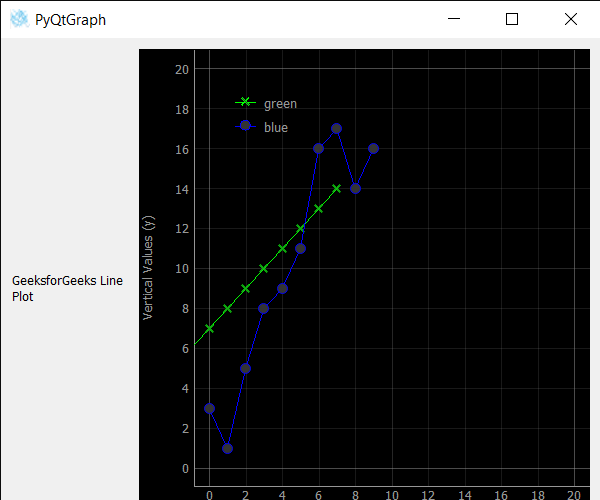Skip to content
Related Articles
PyQtGraph – Setting X and Y Co-ordinates of Line in Line Graph
• Last Updated : 25 Oct, 2020

In this article, we will see how we can set X & Y coordinates of the line i.e origin of the line of line graph in the PyQtGraph module. PyQtGraph is a graphics and user interface library for Python that provides functionality commonly required in designing and science applications. Its primary goals are to provide fast, interactive graphics for displaying data (plots, video, etc.) A line chart or line plot or line graph or curve chart is a type of chart that displays information as a series of data points called ‘markers’ connected by straight line segments. It is a basic type of chart common in many fields. The line graph is created with the help of plot class in PyQtGraph. X and Y are the positions of the line corresponding to the respective axis.
We can create a plot window and create lines on it with the help of commands given below

```# creating a pyqtgraph plot window
plt = pg.plot()

# ploting line in green color
# with dot symbol as x, not a mandatory field
line = plt.plot(x, y, pen='g', symbol='x', symbolPen='g',
symbolBrush=0.2, name='green')
```

In order to do this we use setX and setY methods with the line object
Syntax : line.setX() and line.setY()
Argument : It takes float value as argument
Return : It returns None

Below is the implementation.

## Python3

 `# importing Qt widgets``from` `PyQt5.QtWidgets ``import` `*``import` `sys`` ` `# importing pyqtgraph as pg``import` `pyqtgraph as pg``from` `PyQt5.QtGui ``import` `*`` ` `from` `PyQt5.QtCore ``import` `Qt`` ` ` ` `# Bar Graph class``class` `BarGraphItem(pg.BarGraphItem):`` ` `    ``# constructor which inherit original``    ``# BarGraphItem``    ``def` `__init__(``self``, ``*``args, ``*``*``kwargs):``        ``pg.BarGraphItem.__init__(``self``, ``*``args, ``*``*``kwargs)`` ` `    ``# creating a mouse double click event``    ``def` `mouseDoubleClickEvent(``self``, e):`` ` `        ``# setting scale``        ``self``.setScale(``0.2``)`` ` ` ` `class` `Window(QMainWindow):`` ` `    ``def` `__init__(``self``):``        ``super``().__init__()`` ` `        ``# setting title``        ``self``.setWindowTitle(``"PyQtGraph"``)`` ` `        ``# setting geometry``        ``self``.setGeometry(``100``, ``100``, ``600``, ``500``)`` ` `        ``# icon``        ``icon ``=` `QIcon(``"skin.png"``)`` ` `        ``# setting icon to the window``        ``self``.setWindowIcon(icon)`` ` `        ``# calling method``        ``self``.UiComponents()`` ` `        ``# showing all the widgets``        ``self``.show()`` ` `    ``# method for components``    ``def` `UiComponents(``self``):`` ` `        ``# creating a widget object``        ``widget ``=` `QWidget()`` ` `        ``# creating a new label``        ``label ``=` `QLabel(``"GeeksforGeeks Line Plot"``)`` ` `        ``# making it multiline``        ``label.setWordWrap(``True``)`` ` `        ``# y values to plot by line 1``        ``y ``=` `[``0``, ``1``, ``2``, ``3``, ``4``, ``5``, ``6``, ``7``, ``8``, ``9``]`` ` `        ``# y values to plot by line 2``        ``y2 ``=` `[``3``, ``1``, ``5``, ``8``, ``9``, ``11``, ``16``, ``17``, ``14``, ``16``]``        ``x ``=` `range``(``0``, ``10``)`` ` `        ``# create plot window object``        ``plt ``=` `pg.plot()`` ` `        ``# showing x and y grids``        ``plt.showGrid(x``=``True``, y``=``True``)`` ` `        ``# adding legend``        ``plt.addLegend()`` ` `        ``# set properties of the label for y axis``        ``plt.setLabel(``'left'``, ``'Vertical Values'``, units``=``'y'``)`` ` `        ``# set properties of the label for x axis``        ``plt.setLabel(``'bottom'``, ``'Horizontal Vlaues'``, units``=``'s'``)`` ` `        ``# setting horizontal range``        ``plt.setXRange(``0``, ``20``)`` ` `        ``# setting vertical range``        ``plt.setYRange(``0``, ``20``)`` ` `        ``# ploting line in green color``        ``# with dot symbol as x, not a mandatory field``        ``line1 ``=` `plt.plot(x, y, pen``=``'g'``, symbol``=``'x'``,``                         ``symbolPen``=``'g'``, symbolBrush``=``0.2``, name``=``'green'``)`` ` `        ``# ploting line2 with blue color``        ``# with dot symbol as o``        ``line2 ``=` `plt.plot(x, y2, pen``=``'b'``, symbol``=``'o'``,``                         ``symbolPen``=``'b'``, symbolBrush``=``0.2``, name``=``'blue'``)`` ` `        ``# setting X pos to the line1``        ``line1.setX(``-``2``)`` ` `        ``# setting Y pos of line 1``        ``line1.setY(``5``)`` ` `        ``# label minimum width``        ``label.setMinimumWidth(``120``)`` ` `        ``# Creating a grid layout``        ``layout ``=` `QGridLayout()`` ` `        ``# setting this layout to the widget``        ``widget.setLayout(layout)`` ` `        ``# adding label to the layout``        ``layout.addWidget(label, ``1``, ``0``)`` ` `        ``# plot window goes on right side, spanning 3 rows``        ``layout.addWidget(plt, ``0``, ``1``, ``3``, ``1``)`` ` `        ``# setting this widget as central widget of the main widow``        ``self``.setCentralWidget(widget)`` ` ` ` `# create pyqt5 app``App ``=` `QApplication(sys.argv)`` ` `# create the instance of our Window``window ``=` `Window()`` ` `# start the app``sys.exit(App.``exec``())`

Output:Attention geek! Strengthen your foundations with the Python Programming Foundation Course and learn the basics.

To begin with, your interview preparations Enhance your Data Structures concepts with the Python DS Course. And to begin with your Machine Learning Journey, join the Machine Learning – Basic Level Course

My Personal Notes arrow_drop_up